Developer guides / KerasCV / Custom Image Augmentations with BaseImageAugmentationLayer

# Custom Image Augmentations with BaseImageAugmentationLayer

Author: lukewood
Date created: 2022/04/26
Description: Use BaseImageAugmentationLayer to implement custom data augmentations.View in ColabGitHub source

## Overview

Data augmentation is an integral part of training any robust computer vision model. While KerasCV offers a plethora of prebuild high quality data augmentation techniques, you may still want to implement your own custom technique. KerasCV offers a helpful base class for writing data augmentation layers: `BaseImageAugmentationLayer`. Any augmentation layer built with `BaseImageAugmentationLayer` will automatically be compatible with the KerasCV `RandomAugmentationPipeline` class.

This guide will show you how to implement your own custom augmentation layers using `BaseImageAugmentationLayer`. As an example, we will implement a layer that tints all images blue.

Currently, KerasCV's preprocessing layers only support the TensorFlow backend with Keras 3.

``````!pip install -q —upgrade keras-cv
``````
``````import os

os.environ["KERAS_BACKEND"] = "tensorflow"

import keras
from keras import ops
from keras import layers
import keras_cv
import matplotlib.pyplot as plt
``````

First, let's implement some helper functions for visualization and some transformations.

``````def imshow(img):
img = img.astype(int)
plt.axis("off")
plt.imshow(img)
plt.show()

def gallery_show(images):
images = images.astype(int)
for i in range(9):
image = images[i]
plt.subplot(3, 3, i + 1)
plt.imshow(image.astype("uint8"))
plt.axis("off")
plt.show()

def transform_value_range(images, original_range, target_range):
images = (images - original_range) / (original_range - original_range)
scale_factor = target_range - target_range
return (images * scale_factor) + target_range

def parse_factor(param, min_value=0.0, max_value=1.0, seed=None):
if isinstance(param, keras_cv.core.FactorSampler):
return param
if isinstance(param, float) or isinstance(param, int):
param = (min_value, param)
if param == param:
return keras_cv.core.ConstantFactorSampler(param)
return keras_cv.core.UniformFactorSampler(param, param, seed=seed)
``````

## BaseImageAugmentationLayer Introduction

Image augmentation should operate on a sample-wise basis; not batch-wise. This is a common mistake many machine learning practicioners make when implementing custom techniques. `BaseImageAugmentation` offers a set of clean abstractions to make implementing image augmentation techniques on a sample wise basis much easier. This is done by allowing the end user to override an `augment_image()` method and then performing automatic vectorization under the hood.

Most augmentation techniques also must sample from one or more random distributions. KerasCV offers an abstraction to make random sampling end user configurable: the `FactorSampler` API.

Finally, many augmentation techniques requires some information about the pixel values present in the input images. KerasCV offers the `value_range` API to simplify the handling of this.

In our example, we will use the `FactorSampler` API, the `value_range` API, and `BaseImageAugmentationLayer` to implement a robust, configurable, and correct `RandomBlueTint` layer.

## Overriding `augment_image()`

Let's start off with the minimum:

``````class RandomBlueTint(keras_cv.layers.BaseImageAugmentationLayer):
def augment_image(self, image, *args, transformation=None, **kwargs):
# image is of shape (height, width, channels)
[*others, blue] = ops.unstack(image, axis=-1)
blue = ops.clip(blue + 100, 0.0, 255.0)
return ops.stack([*others, blue], axis=-1)
``````

Our layer overrides `BaseImageAugmentationLayer.augment_image()`. This method is used to augment images given to the layer. By default, using `BaseImageAugmentationLayer` gives you a few nice features for free:

• support for unbatched inputs (HWC Tensor)
• support for batched inputs (BHWC Tensor)
• automatic vectorization on batched inputs (more information on this in automatic vectorization performance)

``````SIZE = (300, 300)
elephants = keras.utils.get_file(
"african_elephant.jpg", "https://i.imgur.com/Bvro0YD.png"
)
elephants = keras.utils.img_to_array(elephants)
imshow(elephants)
``````
``````Downloading data from https://i.imgur.com/Bvro0YD.png
4217496/4217496 ━━━━━━━━━━━━━━━━━━━━ 0s 0us/step
``````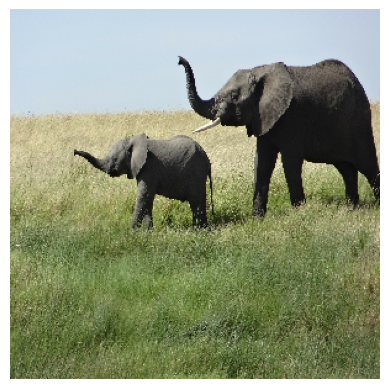Next, let's augment it and visualize the result:

``````layer = RandomBlueTint()
augmented = layer(elephants)
imshow(ops.convert_to_numpy(augmented))
``````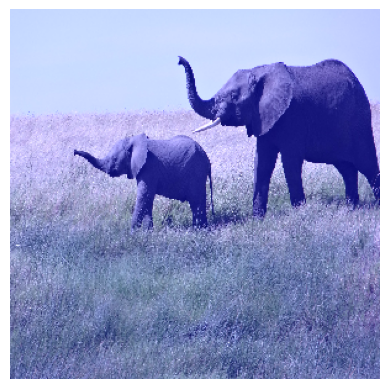Looks great! We can also call our layer on batched inputs:

``````layer = RandomBlueTint()
augmented = layer(ops.expand_dims(elephants, axis=0))
imshow(ops.convert_to_numpy(augmented))
``````## Adding Random Behavior with the `FactorSampler` API.

Usually an image augmentation technique should not do the same thing on every invocation of the layer's `__call__` method. KerasCV offers the `FactorSampler` API to allow users to provide configurable random distributions.

``````class RandomBlueTint(keras_cv.layers.BaseImageAugmentationLayer):
"""RandomBlueTint randomly applies a blue tint to images.

Args:
factor: A tuple of two floats, a single float or a
`keras_cv.FactorSampler`. `factor` controls the extent to which the
image is blue shifted. `factor=0.0` makes this layer perform a no-op
operation, while a value of 1.0 uses the degenerated result entirely.
Values between 0 and 1 result in linear interpolation between the original
image and a fully blue image.
Values should be between `0.0` and `1.0`.  If a tuple is used, a `factor` is
sampled between the two values for every image augmented.  If a single float
is used, a value between `0.0` and the passed float is sampled.  In order to
ensure the value is always the same, please pass a tuple with two identical
floats: `(0.5, 0.5)`.
"""

def __init__(self, factor, **kwargs):
super().__init__(**kwargs)
self.factor = parse_factor(factor)

def augment_image(self, image, *args, transformation=None, **kwargs):
[*others, blue] = ops.unstack(image, axis=-1)
blue_shift = self.factor() * 255
blue = ops.clip(blue + blue_shift, 0.0, 255.0)
return ops.stack([*others, blue], axis=-1)
``````

Now, we can configure the random behavior of ou `RandomBlueTint` layer. We can give it a range of values to sample from:

``````many_elephants = ops.repeat(ops.expand_dims(elephants, axis=0), 9, axis=0)
layer = RandomBlueTint(factor=0.5)
augmented = layer(many_elephants)
gallery_show(ops.convert_to_numpy(augmented))
``````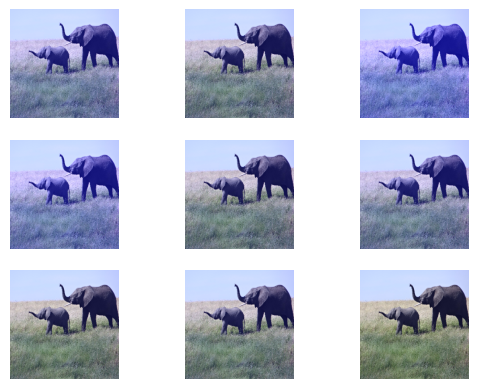Each image is augmented differently with a random factor sampled from the range `(0, 0.5)`.

We can also configure the layer to draw from a normal distribution:

``````many_elephants = ops.repeat(ops.expand_dims(elephants, axis=0), 9, axis=0)
factor = keras_cv.core.NormalFactorSampler(
mean=0.3, stddev=0.1, min_value=0.0, max_value=1.0
)
layer = RandomBlueTint(factor=factor)
augmented = layer(many_elephants)
gallery_show(ops.convert_to_numpy(augmented))
``````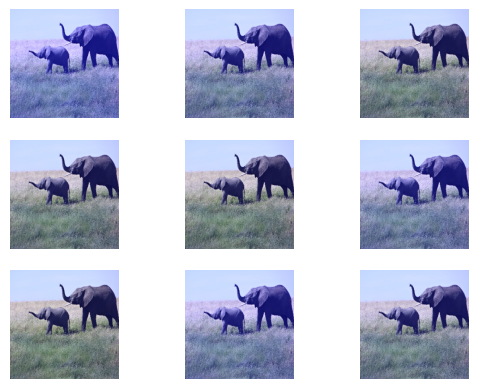As you can see, the augmentations now are drawn from a normal distributions. There are various types of `FactorSamplers` including `UniformFactorSampler`, `NormalFactorSampler`, and `ConstantFactorSampler`. You can also implement you own.

## Overridding `get_random_transformation()`

Now, suppose that your layer impacts the prediction targets: whether they are bounding boxes, classification labels, or regression targets. Your layer will need to have information about what augmentations are taken on the image when augmenting the label. Luckily, `BaseImageAugmentationLayer` was designed with this in mind.

To handle this issue, `BaseImageAugmentationLayer` has an overrideable `get_random_transformation()` method alongside with `augment_label()`, `augment_target()` and `augment_bounding_boxes()`. `augment_segmentation_map()` and others will be added in the future.

Let's add this to our layer.

``````class RandomBlueTint(keras_cv.layers.BaseImageAugmentationLayer):
"""RandomBlueTint randomly applies a blue tint to images.

Args:
factor: A tuple of two floats, a single float or a
`keras_cv.FactorSampler`. `factor` controls the extent to which the
image is blue shifted. `factor=0.0` makes this layer perform a no-op
operation, while a value of 1.0 uses the degenerated result entirely.
Values between 0 and 1 result in linear interpolation between the original
image and a fully blue image.
Values should be between `0.0` and `1.0`.  If a tuple is used, a `factor` is
sampled between the two values for every image augmented.  If a single float
is used, a value between `0.0` and the passed float is sampled.  In order to
ensure the value is always the same, please pass a tuple with two identical
floats: `(0.5, 0.5)`.
"""

def __init__(self, factor, **kwargs):
super().__init__(**kwargs)
self.factor = parse_factor(factor)

def get_random_transformation(self, **kwargs):
# kwargs holds {"images": image, "labels": label, etc...}
return self.factor() * 255

def augment_image(self, image, transformation=None, **kwargs):
[*others, blue] = ops.unstack(image, axis=-1)
blue = ops.clip(blue + transformation, 0.0, 255.0)
return ops.stack([*others, blue], axis=-1)

def augment_label(self, label, transformation=None, **kwargs):
# you can use transformation somehow if you want

if transformation > 100:
# i.e. maybe class 2 corresponds to blue images
return 2.0

return label

def augment_bounding_boxes(self, bounding_boxes, transformation=None, **kwargs):
# you can also perform no-op augmentations on label types to support them in
return bounding_boxes
``````

To make use of these new methods, you will need to feed your inputs in with a dictionary maintaining a mapping from images to targets.

As of now, KerasCV supports the following label types:

• labels via `augment_label()`.
• bounding_boxes via `augment_bounding_boxes()`.

In order to use augmention layers alongside your prediction targets, you must package your inputs as follows:

``````labels = ops.array([[1, 0]])
inputs = {"images": ops.convert_to_tensor(elephants), "labels": labels}
``````

Now if we call our layer on the inputs:

``````layer = RandomBlueTint(factor=(0.6, 0.6))
augmented = layer(inputs)
print(augmented["labels"])
``````
``````2.0
``````

Both the inputs and labels are augmented. Note how when `transformation` is > 100 the label is modified to contain 2.0 as specified in the layer above.

## `value_range` support

Imagine you are using your new augmentation layer in many pipelines. Some pipelines have values in the range `[0, 255]`, some pipelines have normalized their images to the range `[-1, 1]`, and some use a value range of `[0, 1]`.

If a user calls your layer with an image in value range `[0, 1]`, the outputs will be nonsense!

``````layer = RandomBlueTint(factor=(0.1, 0.1))
elephants_0_1 = elephants / 255
print("min and max before augmentation:", elephants_0_1.min(), elephants_0_1.max())
augmented = layer(elephants_0_1)
print(
"min and max after augmentation:",
ops.convert_to_numpy(augmented).min(),
ops.convert_to_numpy(augmented).max(),
)
imshow(ops.convert_to_numpy(augmented * 255).astype(int))
``````
``````Clipping input data to the valid range for imshow with RGB data ([0..1] for floats or [0..255] for integers).

min and max before augmentation: 0.0 1.0
min and max after augmentation: 0.0 26.488235
``````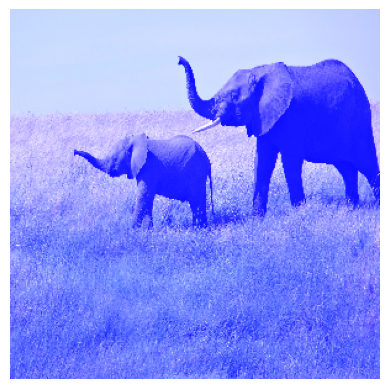Note that this is an incredibly weak augmentation! Factor is only set to 0.1.

Let's resolve this issue with KerasCV's `value_range` API.

``````class RandomBlueTint(keras_cv.layers.BaseImageAugmentationLayer):
"""RandomBlueTint randomly applies a blue tint to images.

Args:
value_range: value_range: a tuple or a list of two elements. The first value
represents the lower bound for values in passed images, the second represents
the upper bound. Images passed to the layer should have values within
`value_range`.
factor: A tuple of two floats, a single float or a
`keras_cv.FactorSampler`. `factor` controls the extent to which the
image is blue shifted. `factor=0.0` makes this layer perform a no-op
operation, while a value of 1.0 uses the degenerated result entirely.
Values between 0 and 1 result in linear interpolation between the original
image and a fully blue image.
Values should be between `0.0` and `1.0`.  If a tuple is used, a `factor` is
sampled between the two values for every image augmented.  If a single float
is used, a value between `0.0` and the passed float is sampled.  In order to
ensure the value is always the same, please pass a tuple with two identical
floats: `(0.5, 0.5)`.
"""

def __init__(self, value_range, factor, **kwargs):
super().__init__(**kwargs)
self.value_range = value_range
self.factor = parse_factor(factor)

def get_random_transformation(self, **kwargs):
# kwargs holds {"images": image, "labels": label, etc...}
return self.factor() * 255

def augment_image(self, image, transformation=None, **kwargs):
image = transform_value_range(image, self.value_range, (0, 255))
[*others, blue] = ops.unstack(image, axis=-1)
blue = ops.clip(blue + transformation, 0.0, 255.0)
result = ops.stack([*others, blue], axis=-1)
result = transform_value_range(result, (0, 255), self.value_range)
return result

def augment_label(self, label, transformation=None, **kwargs):
# you can use transformation somehow if you want

if transformation > 100:
# i.e. maybe class 2 corresponds to blue images
return 2.0

return label

def augment_bounding_boxes(self, bounding_boxes, transformation=None, **kwargs):
# you can also perform no-op augmentations on label types to support them in
return bounding_boxes

layer = RandomBlueTint(value_range=(0, 1), factor=(0.1, 0.1))
elephants_0_1 = elephants / 255
print("min and max before augmentation:", elephants_0_1.min(), elephants_0_1.max())
augmented = layer(elephants_0_1)
print(
"min and max after augmentation:",
ops.convert_to_numpy(augmented).min(),
ops.convert_to_numpy(augmented).max(),
)
imshow(ops.convert_to_numpy(augmented * 255).astype(int))
``````
``````min and max before augmentation: 0.0 1.0
min and max after augmentation: 0.0 1.0
``````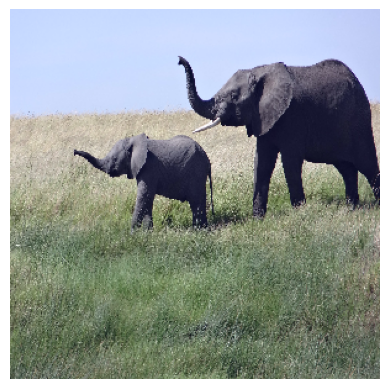Now our elephants are only slgihtly blue tinted. This is the expected behavior when using a factor of `0.1`. Great!

Now users can configure the layer to support any value range they may need. Note that only layers that interact with color information should use the value range API. Many augmentation techniques, such as `RandomRotation` will not need this.

## Auto vectorization performance

If you are wondering:

Does implementing my augmentations on an sample-wise basis carry performance implications?

You are not alone!

Luckily, I have performed extensive analysis on the performance of automatic vectorization, manual vectorization, and unvectorized implementations. In this benchmark, I implemented a RandomCutout layer using auto vectorization, no auto vectorization and manual vectorization. All of these were benchmarked inside of an `@tf.function` annotation. They were also each benchmarked with the `jit_compile` argument.

The following chart shows the results of this benchmark:The primary takeaway should be that the difference between manual vectorization and automatic vectorization is marginal!

Please note that Eager mode performance will be drastically different.

## Common gotchas

Some layers are not able to be automatically vectorizated. An example of this is GridMask.

``````class UnVectorizable(keras_cv.layers.BaseImageAugmentationLayer):
def __init__(self, **kwargs):
super().__init__(**kwargs)
# this disables BaseImageAugmentationLayer's Auto Vectorization
self.auto_vectorize = False
``````

Additionally, be sure to accept `**kwargs` to your `augment_*` methods to ensure forwards compatibility. KerasCV will add additional label types in the future, and if you do not include a `**kwargs` argument your augmentation layers will not be forward compatible.

## Conclusion and next steps

KerasCV offers a standard set of APIs to streamline the process of implementing your own data augmentation techniques. These include `BaseImageAugmentationLayer`, the `FactorSampler` API and the `value_range` API.

We used these APIs to implement a highly configurable `RandomBlueTint` layer. This layer can take inputs as standalone images, a dictionary with keys of `"images"` and labels, inputs that are unbatched, or inputs that are batched. Inputs may be in any value range, and the random distribution used to sample the tint values is end user configurable.

As a follow up exercises you can: